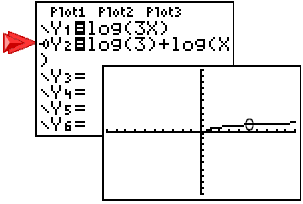Working with Logarithms

A logarithm is an exponent.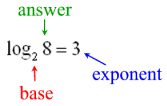As this example shows, 3 is the exponent to which the base 2 must be raised to create the answer of 8,
or  23 = 8.  In general terms: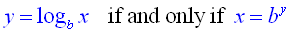(where x > 0 and b is a positive constant not equal to 1)
 BASE 10:Logarithms with base 10 are called common logarithms. When the base is not indicated, base 10 is implied. The log key on the graphing calculator will calculate the common (or base 10) logarithm. 2nd log will calculate the antilogarithm or 10x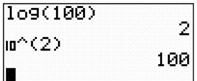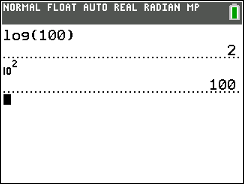OTHER BASES: To enter a logarithm with a different base on the graphing calculator, use the Change of Base Formula: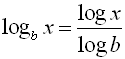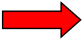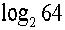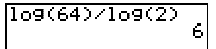If your calculator has the logBASE operation template, you can enter the values as they appear in the expression. Go to: MATH → arrow down to A:logBASE( Or hit MATH, ALPHA (key), MATH to get to the A: option.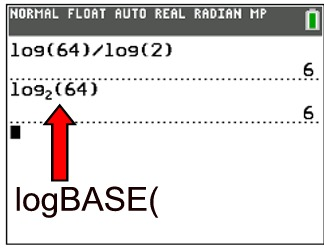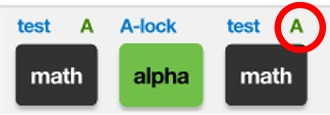You can also hit ALPHA (key), WINDOW and choose the fifth option on the menu, logBASE(.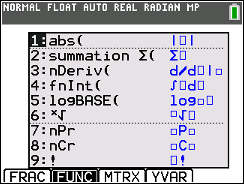BASE e:

Logarithms with base e are called natural logarithms.
Natural logarithms are denoted by ln.
On the graphing calculator, the base e logarithm is the ln key.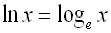e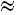2.71828183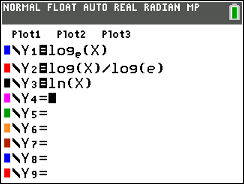All three are the same.

When working with logarithms on your graphing calculator,
you must remember the "Change of Base Formula":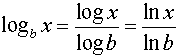Remember, the notation:  log x is with respect to base 10 ln x is with respect to base e

Examples:

1.  Evaluate: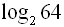2.  Evaluate:  ln 2;  ln 1;  ln e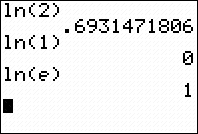3.  Evaluate: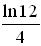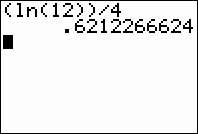4.  Sketch the graph of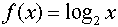If you have the logBASE function, it can be used to enter the function (seen in Y1 below).
If not, use the Change of Base formula (see in Y2 below).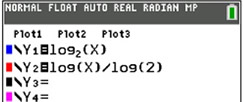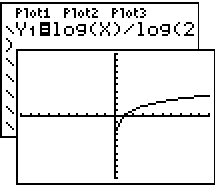5.  Use a graph to support the conclusion that the inverse of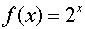is.

A function composed with its inverse creates the identity line y = x.  Showing that the composition of these two functions is the identity line shows that these functions are inverses of one another.  Note the bubble animation set for Y4 to show that the linear lines in Y3 and Y4 are equivalent.
You are showing that the composition of Y1(Y2) = x (the Identity Function).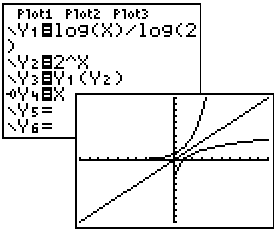6.  Solve graphically: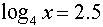Method 2:  This problem can also be solved by setting the equation equal to 0 and finding the x-intercepts, or zeros (2nd CALC, #2), of the function.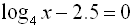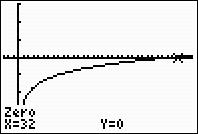Method 1: Graph each side separately.
The window must be adjusted to show a sufficient amount of the x-axis to locate the intersection point (2nd CALC, #5).  The answer is x = 32.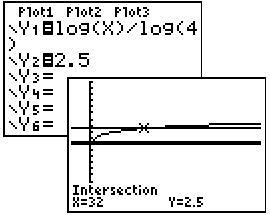7.  Using your graphing calculator, determine which of the following statements are true.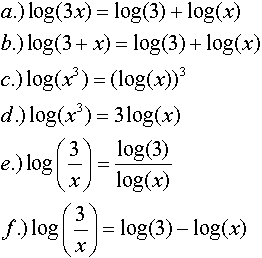a.) T  b.) F  c.) F  d.) T  e.) F  f.)  T
Solution for part a:  (TRUE)
Place the left side of the equation in Y1 and the right side in Y2.  Turn on the "bubble animation" to the left of the Y2.  This will allow you to see the bubble floating over the graph when the equation is true.  Parts b - f are solved in a similar manner.